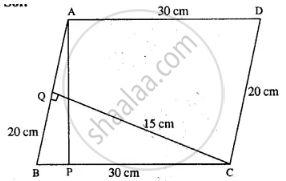# A Parallelogram Has Sides of 20 Cm and 30 Cm. If the Distance Between Its Shorter Sides is 15 Cm; Find the Distance Between the Longer Sides. - Mathematics

Sum

A parallelogram has sides of 20 cm and 30 cm. If the distance between its shorter sides is 15 cm; find the distance between the longer sides.

#### SolutionLet ABCD be the ||gm in which BC = 30 cm

and CD = 20 cm

Distance between shorter sides,

i.e. CQ = 15 cm

∴ area of ||gm = AB × CQ

= 20 × 15

= 300 cm2

Again BC × AP = Area of || gm

30 × AP = 300

AP = 300/30

AP = 10 cm

∴ Distance between larger sides is = 10 cm

Concept: Area of a Parallelogram
Is there an error in this question or solution?

#### APPEARS IN

Selina Concise Mathematics Class 8 ICSE
Chapter 20 Area of a Trapezium and a Polygon
Exercise 20 (C) | Q 5 | Page 232